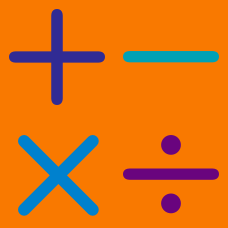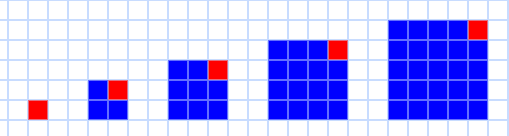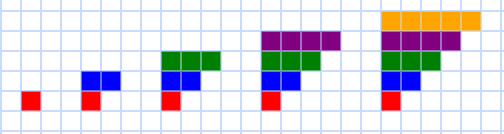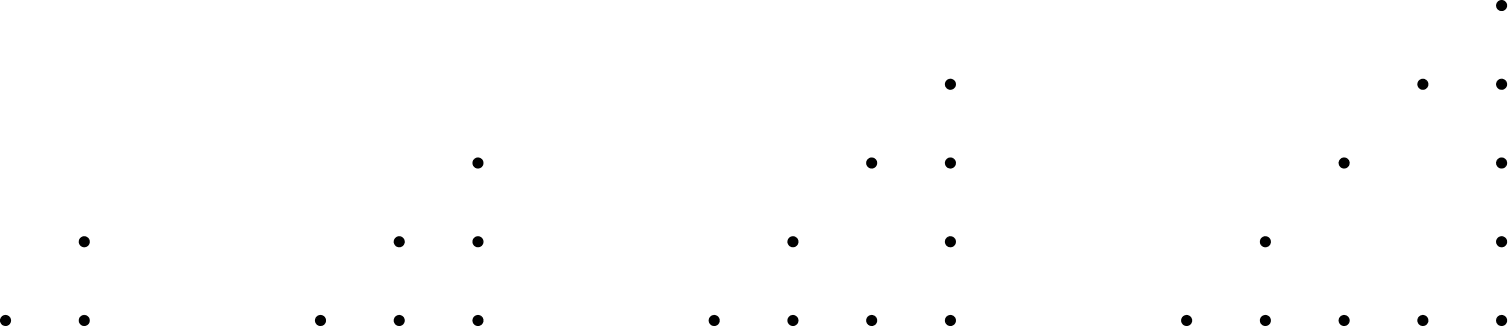Everyday Math

# Numerical Patterns Warmup$0, 3, 8, 15, 24, \ldots$ What is the next number in this sequence?$1, 3, 6, 10, 15, \ldots$ What is the next number in this sequence?How many dots will there be in the next picture in this sequence?

The prime numbers (numbers divisible by only 1 and themselves) follow the sequence: 2, 3, 5, 7, 11, 13, 17, 19, 23, 29, 31, 37, ...

Given that, consider: $5, 8, 12, 18, 24, \ldots$ What is the next number in this sequence?

$20, 17, 14, 11, 8, \ldots$ What is the next number in this sequence?

×